Side Side Angle Calculator To be used only with side-side-non-included angle triangles. For all other triangles, click here.
************************************************
Side Side Angle Calculator
Angles Are In or

>>>>>
>>>>>
>>>>>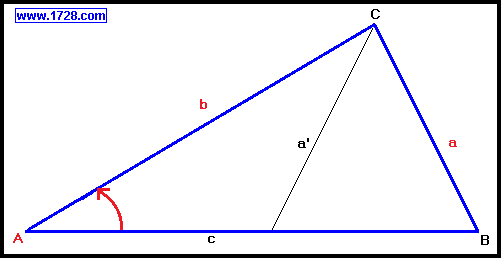The above diagram shows a typical case of solving a triangle when we are given two sides and one non-included angle.
Here, we are given side b, side a and its opposing angle A.

The number of solutions we will get depends upon the length of side a compared to the height, which is determined by this formula:

height (or side a) = side b • sine (angle A)

and so if: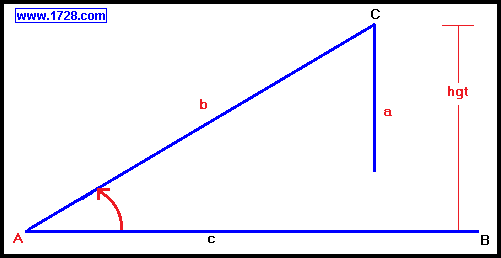side a < height - no solution because side a doesn't "reach" side c.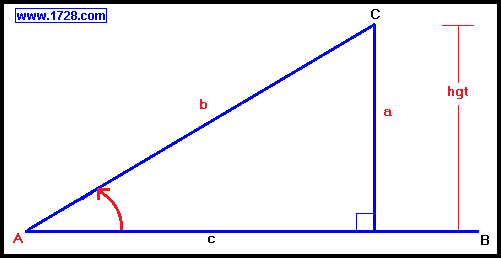side a = height - one solution.
Side a just "reaches" side c and forms a right triangle.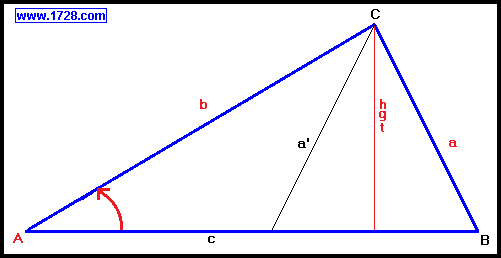side a > height - two solutions.
This is the ambiguous case. Side a is long enough to reach side c in two places.
For this to occur, side a has to be greater than the height but less than side c.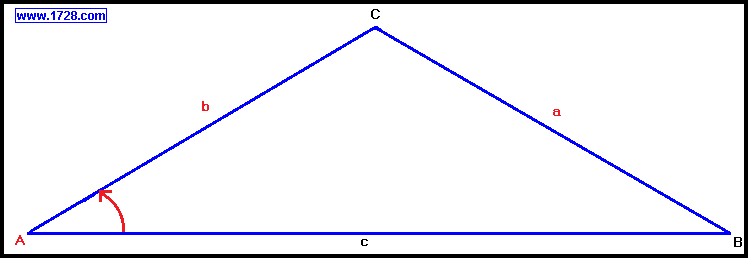side a >= side b - one solution.
Side a is now so long that it can only intersect the triangle at point B.
Unlike the ambiguous case, there can't be a matching "left side" (like side a prime) because side a is so long, it can no longer intersect the left-side of side c.

Significant Figures >>>
Numbers are displayed in scientific notation with the amount of significant figures you specify. For easier readability, numbers between .001 and 1,000 will not be in scientific notation but will still have the same precision.
You may change the number of significant figures displayed by changing the number in the box above.
Most browsers, will display the answers properly but if you are seeing no answers at all, enter a zero in the box above, which will eliminate all formatting but at least you will see the answers.